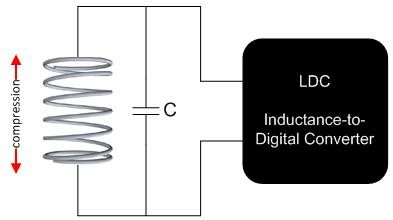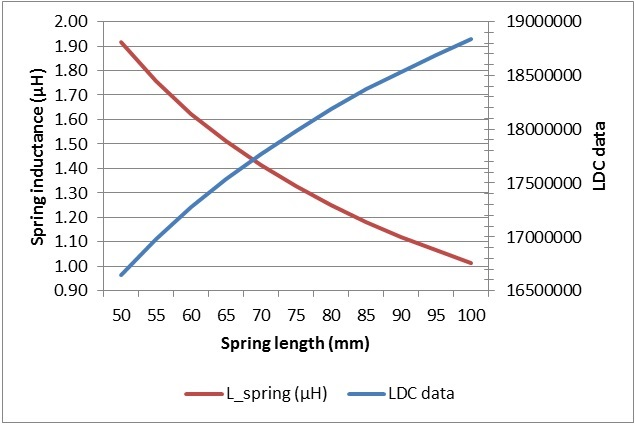# Inductive sensing: How to sense spring compression

While most inductive-sensing applications use either printed circuit board (PCB) coils or wire-wound inductors as the sensor, inductance-to-digital converters (LDCs) can use almost any inductor as a sensor – even a spring. Springs are useful as sensors because the spring’s inductance varies directly with changes in length or other physical changes. Figure 1 shows how to connect a spring to an LDC.Figure 1: Spring used as a sensor by an LDC

To evaluate using a spring as a sensor, I used the LDC1612EVM evaluation module to measure the inductance of a spring as I extended the spring across a range of lengths. To do this, I first removed the on-board sensor from the EVM and replaced it with a spring. The spring was made of 0.7mm-thick steel, had 46 turns and a diameter of 7.3mm. Figure 2 shows the spring that I connected to the EVM.

Figure 2: Spring setup

The inductance of my spring is too low to be used as a sensor for the LDC1612 on its own, so I added a 2.2μH fixed wire-wound surface-mount device (SMD) inductor in series. (For details on how to use a series inductor to increase sensor impedance, see my blog post “How to use a tiny 2mm PCB inductor as a sensor.”) With a 1nF sensor capacitor, the oscillation frequency was 2.5MHz. Figure 3 shows the sensor components that I used.

Figure 3: Sensor components

I stretched the spring from 50mm to 100mm in 5mm increments and measured LDC1612 output data at each step. From the data, I calculated the sensor inductance using Equation 1:

where

and fref = reference clock (40MHz on the LDC1612 EVM).

Figure 4 shows the data and spring inductance after subtracting the 2.2μH series inductor.Figure 4: LDC1612 data and spring inductance versus spring length

The data samples that I collected when extending the spring from 50mm to 100mm in 5mm steps are monotonic and can be used to precisely determine the length of the spring. During this spring-compression range, the inductance decreases from 1.92μH (LDC output 16,644,000) to 1.01μH (LDC output 18,840,000). Thus, over this range, stretching the spring by 1μm results in a 44-codes increment in the LDC1612 data output on average.

This data shows that you can use inductive sensing to directly measure the inductance shift that results from compressing a spring, and that springs can serve as an alternative sensor to PCB coils and wire-wound inductors.

• Hi,

I have similar application to change in Inductance value of a spring after its movement (expansion/compression).

The springs L = 3µH, Series resistance Rs = 3Ω. If I use C = 1nF, which means; Resonant Fr = 2.9MHz and Rp = 1K.
It is required to measure change in Inductance of 0.01µH (10nH).

1. Would LDC1612 be able to detect this?
2. How to optimize resolution to detect change in Inductance with LDC1612, i.e. Increase C to 2.2nF ? Add additional inductance on 2.2µH in series with spring?
• Hi,

Could you share how to calculate L(inductance) of your spring?

Regards,

y.i